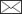Aarne Nyyssönen, Pekka Kilkki, Erkki Mikkola

Eräiden metsänarvioimismenetelmien tarkkuudesta

Nyyssönen A., Kilkki P., Mikkola E. (1967). Eräiden metsänarvioimismenetelmien tarkkuudesta. Acta Forestalia Fennica vol. 81 no. 4 article id 7169. https://doi.org/10.14214/aff.7169

English title: On the precision of some methods of forest inventory

Abstract

This paper reports on tests made for the study of alternative methods in forest survey. Data were acquired by measurements in five areas in Finland and in Mexico, varying in size from 20 to 900 ha. The principal characteristics used in the analysis was the entire volume. By the combination of neighbouring plots, the variation could be studied for different plot sizes and survey strips. Variable (relascope) plots could be compared.

A starting point for the comparison of different sampling methods, calculations were made of the coefficients of variation for each plot type; total and within the strata. The amount of decrease of variation with an increasing plot size could be established. Comparisons have been made of the following sampling methods: simple random, stratified random, simple systematic, and stratified systematic sampling.

On comparisons of the standard error of sample mean it was found that in both stratified sampling and different types of systematic sampling there is, with increasing size and diminishing interval of sample plots, an increase in the relative improvement of the result against simple random sampling. Only in exceptional cases did systematic surveys give results which were less precise than those derived by other methods.

In discussion of some methods for determination of the precision of systematic sampling, possibilities of theoretical determination of the degree of precision was considered. An empirical study was made of the behaviour of some equations based on the sample itself. The larger the plot size and the shorter the plot interval, the more the equations overestimated in general the variance of sample mean.

As none of the equations studied gave reliable results, regression equations were calculated for the relative standard error on the basis of the data measured. The independent variables were plot size, plot or strip interval, area of survey unit and mean volume. The results arrived at are based mainly on the complete measurement of one area only. To enable extension of the scope of application, more material is needed with a complete enumeration of trees.

The PDF includes a summary in Finnish.

Published in 1967

Views 682

Available at https://doi.org/10.14214/aff.7169 |Register
Click this link to register to Silva Fennica.